# 关于.NET调用原生Python

会研究这个课题纯粹是因为尝试一下能否实现。其实关于.NET调用Python，已经有了IronPython，但是IronPython的更新缓慢，库安装也不如原生Python那么方便。（大佬可以无视我），如果能实现.NET调用Python，那么两者混合编程开发将会提高效率，在某些特殊的应用场景中。

其实混合编程方案众多，例如开发本地服务器，或者利用文件来进行交互。但是一来速度慢，二来不够安全。虽然很多会这样实现吧。我认为Python也提供了更加安全的方式。

原理大家都懂，Python是C开发的，所以通过C调用Python，然后在用托管C++开发CLR类库给C#调用。这样，我们只需要对Python源码和CLI有一定的认识和了解就可以做到了。并不是什么难事，以下是我个人的开发过程

首先我们尝试使用C++来调用Python，网上教程很多。我新建了一个C++空项目。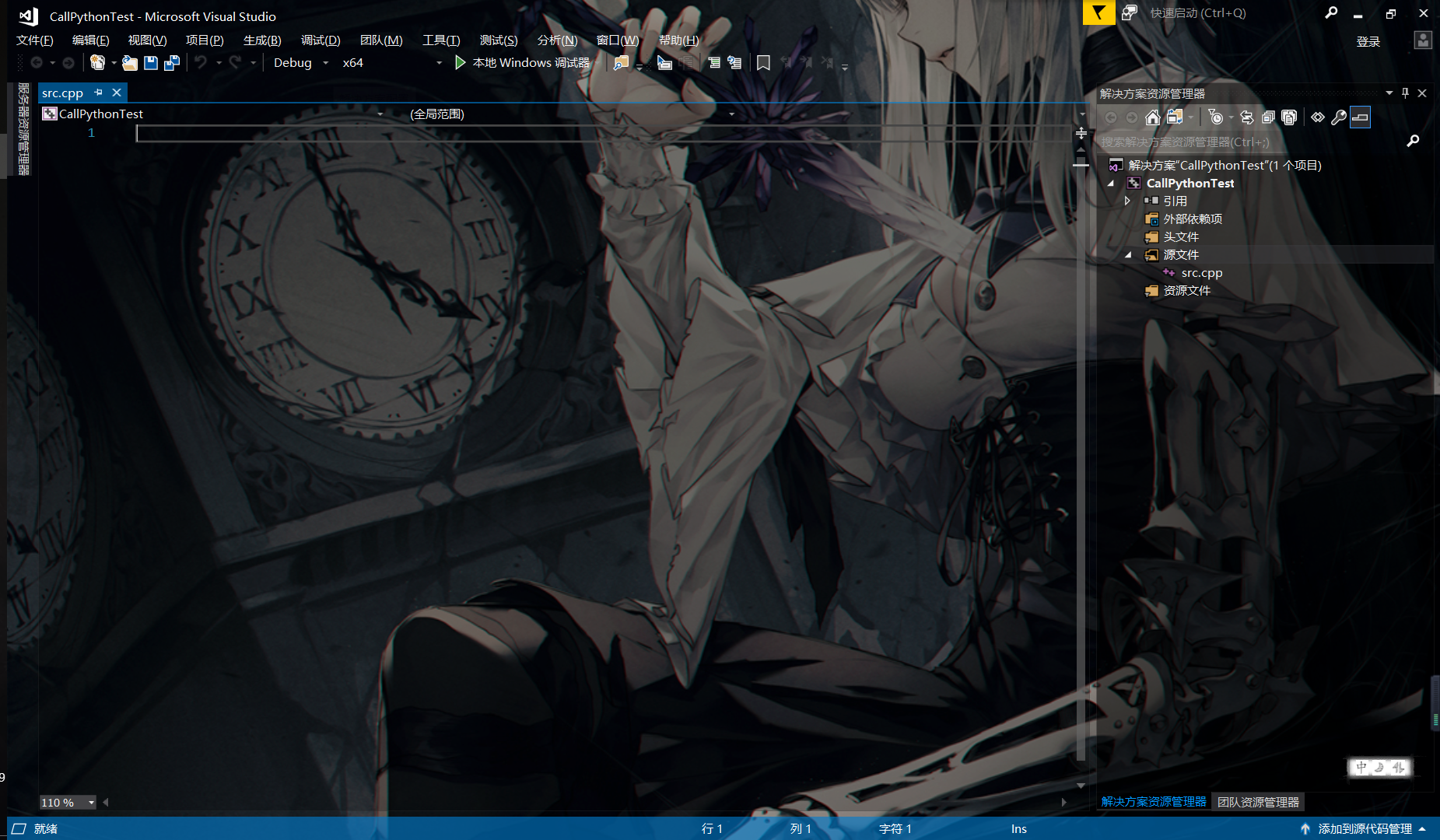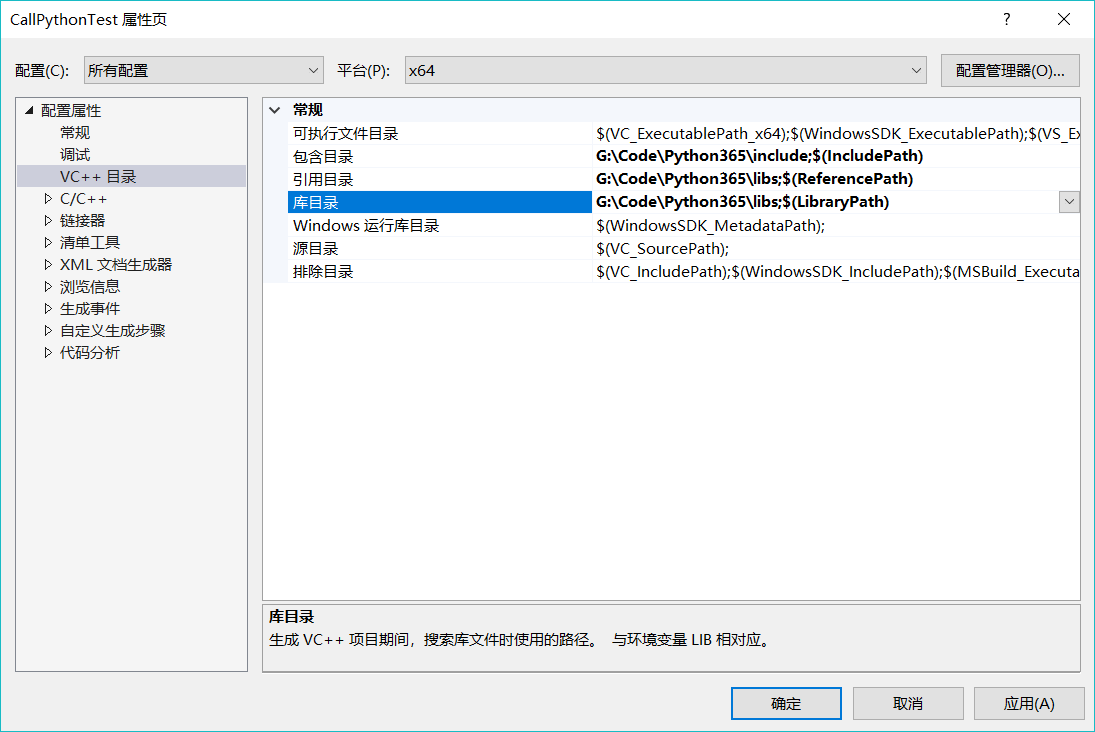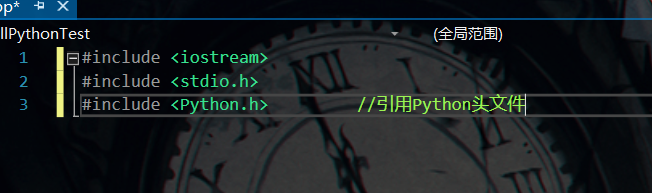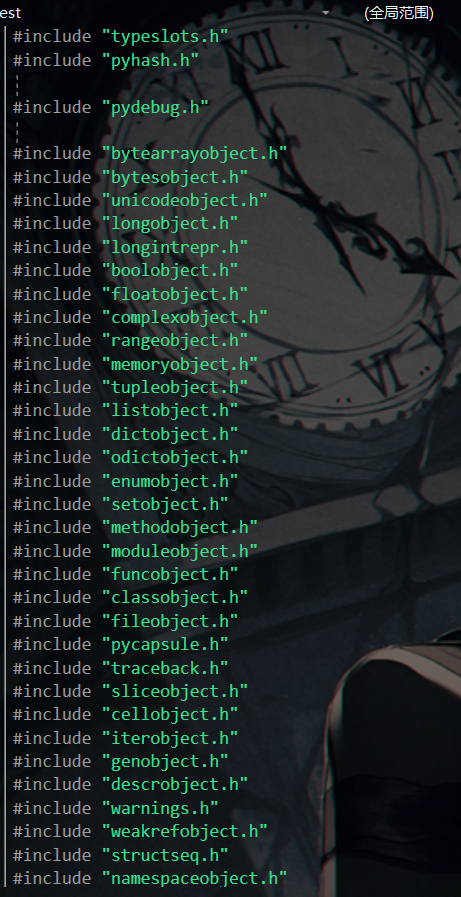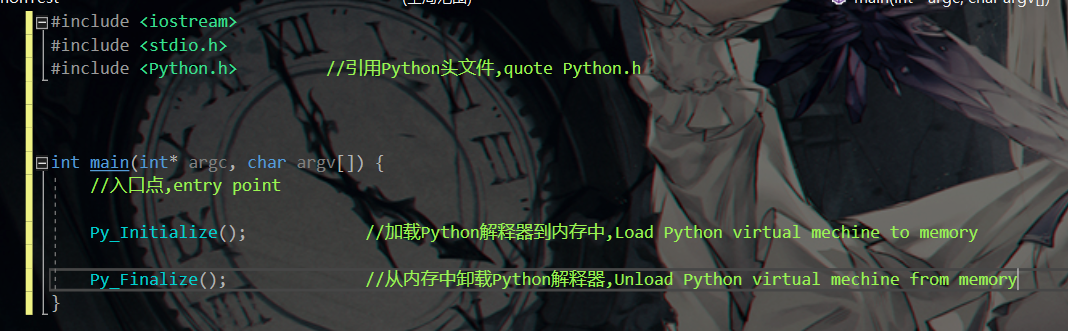#include <iostream>
#include <stdio.h>
#include <Python.h>			//引用Python头文件,quote Python.h

int main(int* argc, char argv[]) {
//入口点,entry point

Py_Initialize();			//加载Python解释器到内存中,Load Python virtual mechine to memory
if (Py_IsInitialized()) {
std::cout << "成功加载Python解释器" << std::endl;
}
else {
std::cout << "未成功加载Python解释器" << std::endl;
}

Py_Finalize();				//从内存中卸载Python解释器,Unload Python virtual mechine from memory
getchar();
return 1;
}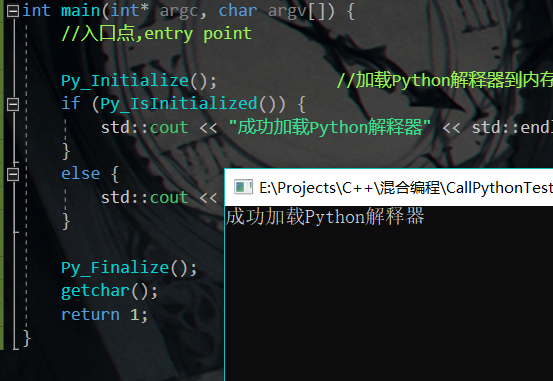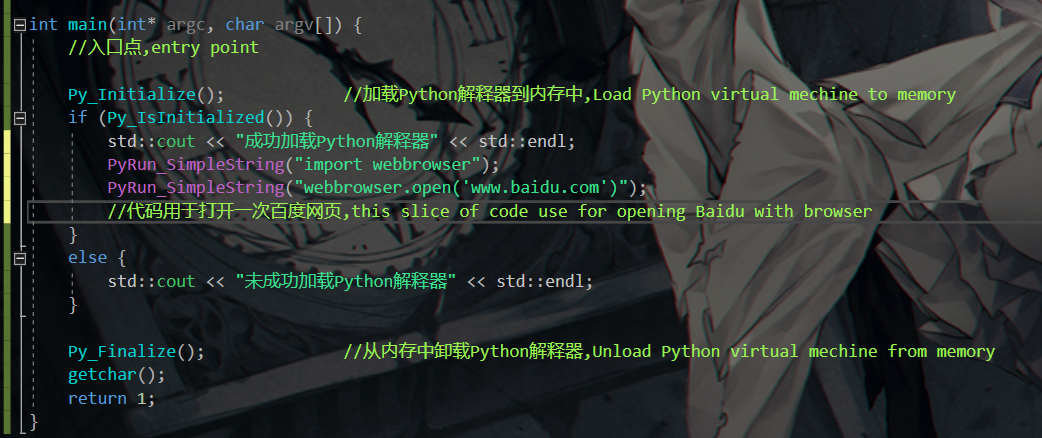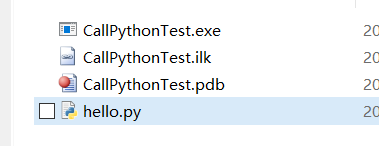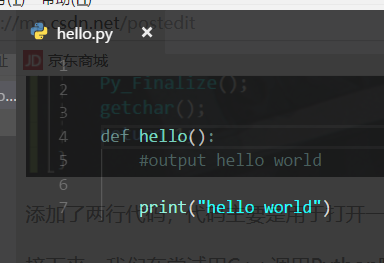#include <iostream>
#include <stdio.h>
#include <Python.h>			//引用Python头文件,quote Python.h

int main(int* argc, char argv[]) {
//入口点,entry point

Py_Initialize();			//加载Python解释器到内存中,Load Python virtual mechine to memory
if (Py_IsInitialized()) {
std::cout << "成功加载Python解释器" << std::endl;

PyObject* PyMod = PyImport_ImportModule("hello");
//导入hello模块，类似于python中的import
//import hello module,just like 'import module' in Python code
if (PyMod) {
//检测是否成功的导入了模块,check if PyMod is valid

PyObject* PyFunc = PyObject_GetAttrString(PyMod, "hello");

//从PyMod中获得hello函数,这是一个Python中的深度魔法
//Obtain hello function from PyMod,and this is a deep magic in Python

if (PyFunc) {
//检测是否成功的引入了函数,check if PyFunc is valid

PyObject_CallObject(PyFunc, NULL);

//执行函数,call function
}
else {
std::cout << "没有成功的引入Python函数" << std::endl;
}
}
else {
std::cout << "没有成功的引入Python模块" << std::endl;
}

}
else {
std::cout << "未成功加载Python解释器" << std::endl;
}

Py_Finalize();				//从内存中卸载Python解释器,Unload Python virtual mechine from memory
getchar();
return 1;
}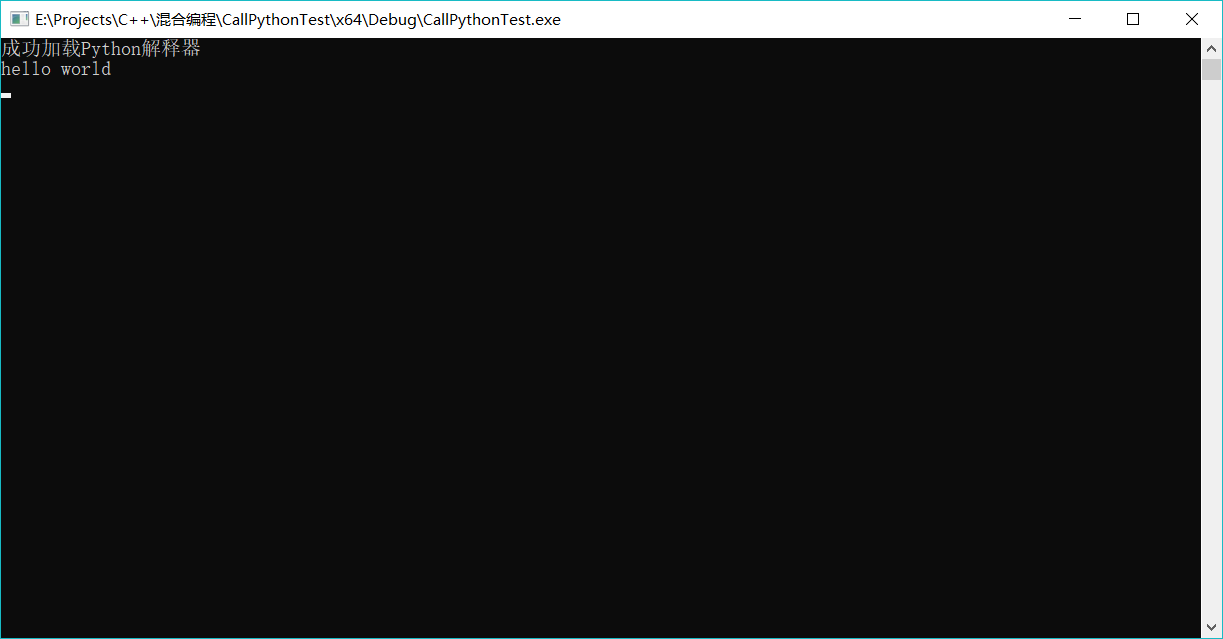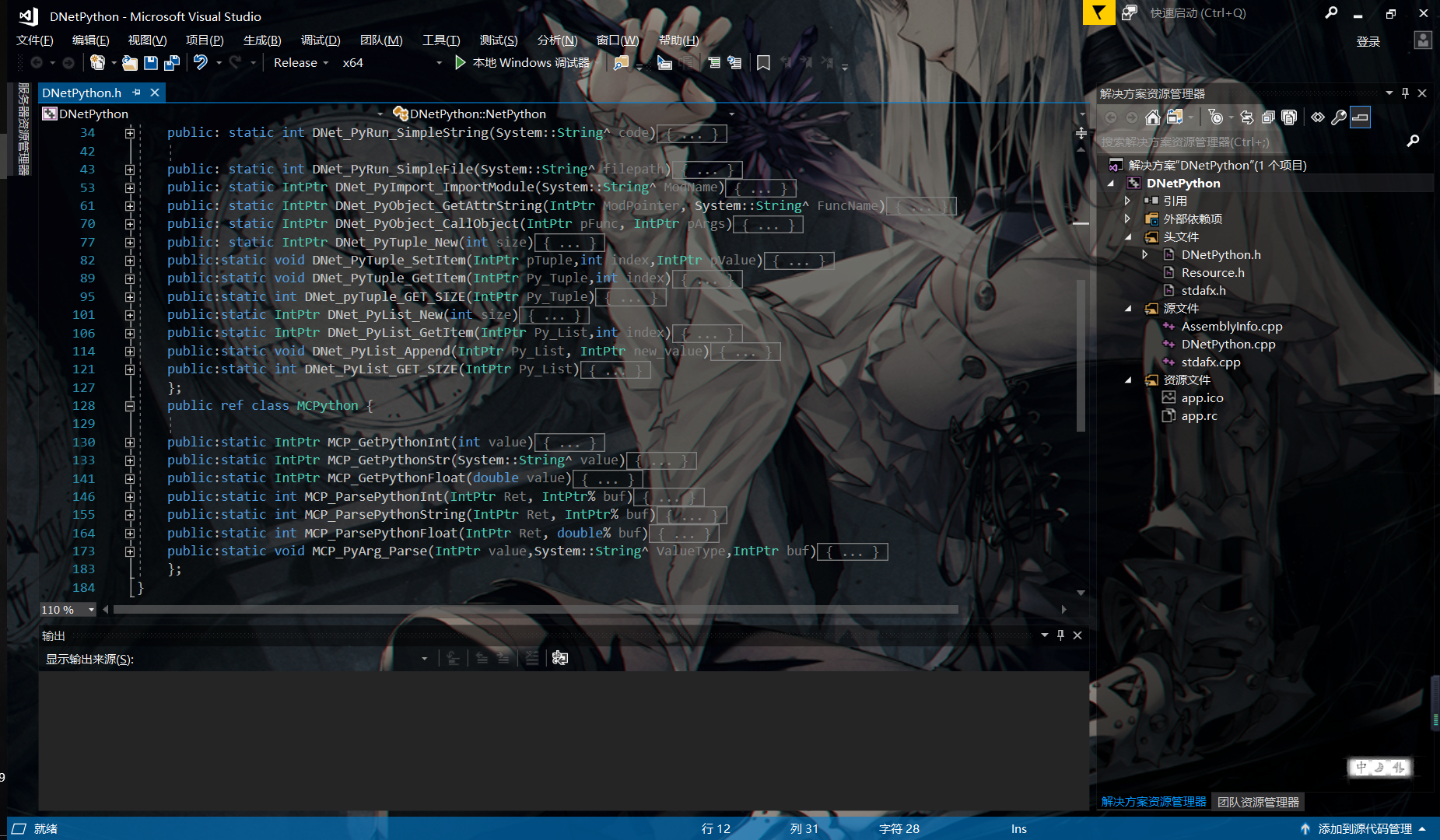https://download.csdn.net/download/f529324416/10382724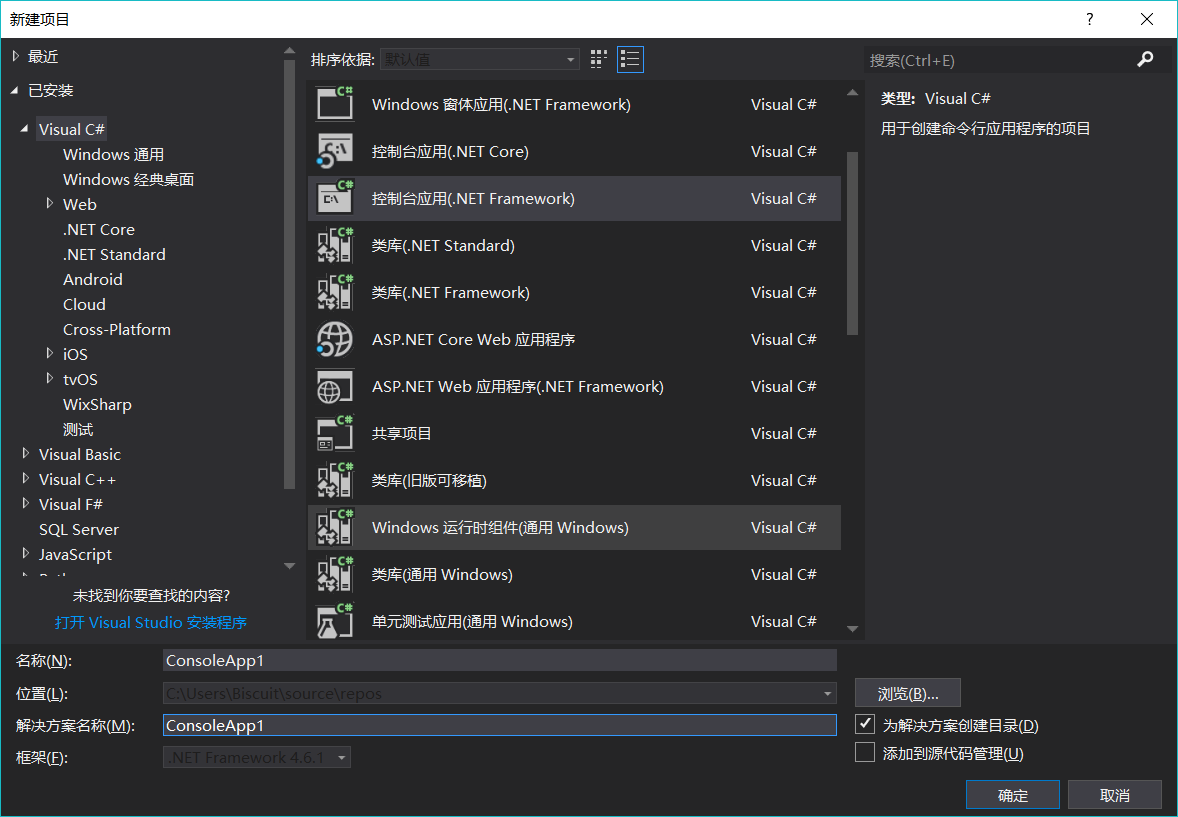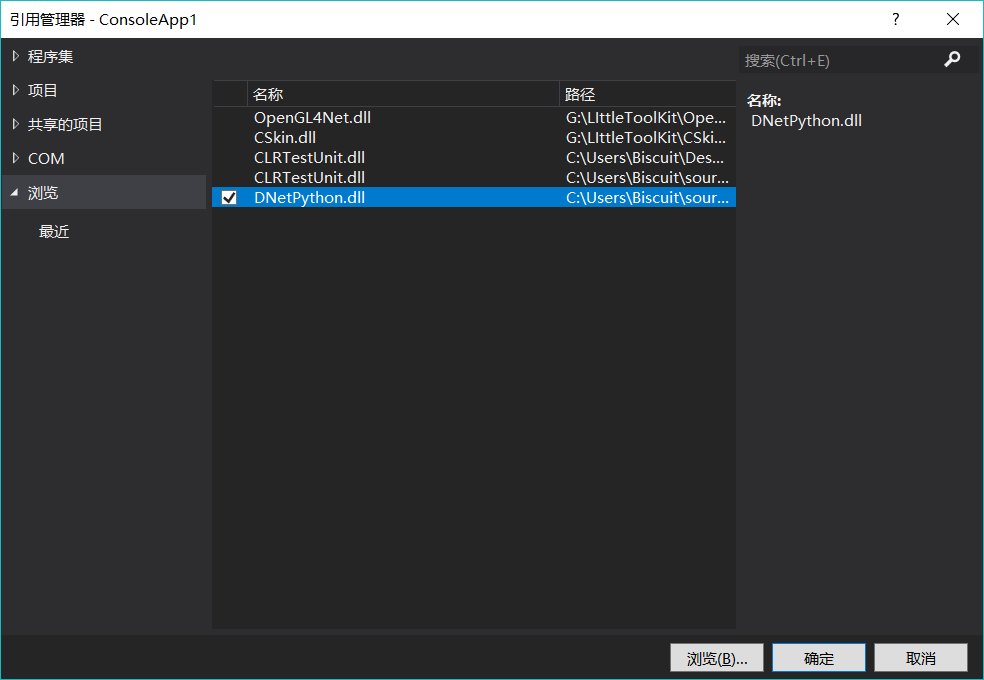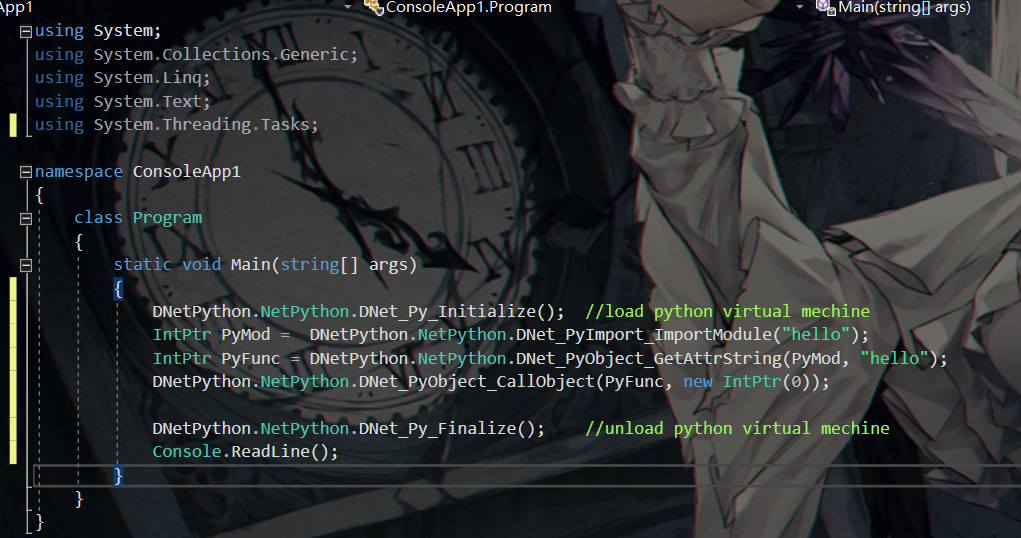using System;
using System.Collections.Generic;
using System.Linq;
using System.Text;
using System.Threading.Tasks;

namespace ConsoleApp1
{
class Program
{
static void Main(string[] args)
{
DNetPython.NetPython.DNet_Py_Initialize();  //load python virtual mechine
IntPtr PyMod =  DNetPython.NetPython.DNet_PyImport_ImportModule("hello");
IntPtr PyFunc = DNetPython.NetPython.DNet_PyObject_GetAttrString(PyMod, "hello");
DNetPython.NetPython.DNet_PyObject_CallObject(PyFunc, new IntPtr(0));

DNetPython.NetPython.DNet_Py_Finalize();    //unload python virtual mechine
Console.ReadLine();
}
}
}